Sie sind hier: ICP » R. Hilfer » Publikationen

# 9 Irreversibility

[216.1.1] The result in eq. (27) has provided new insight into the irreversibility paradox [21, p. 554]. [216.1.2] For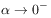one finds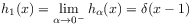(30)

and therefore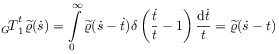(31)

is a right translation. [216.1.3] Here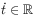denotes a time instant, while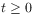is a time duration. [216.1.4] This shows, that induced right translations do not form a group, but only a semigroup.

[page 217, §1]    [217.1.1] These observations suggest a reformulation of the controversial irreversibility problem [49, 6]. [217.1.2] The problem of irreversibility is normally formulated as:

###### Definition 9.1 (The normal irreversibility problem).

[217.1.3] Assume that time is reversible. Explain how and why time irreversible equations arise in physics.

[217.1.4] The assumption that time is reversible, i.e., is made in all fundamental theories of modern physics. [217.1.5] The explanation of macroscopically irreversible behaviour for macroscopic nonequilibrium states of subsystems is due to Boltzmann. [217.1.6] It is based on the applicability of statistical mechanics and thermodynamics, the large separation of scales, the importance of low entropy initial conditions, and probabilistic reasoning .

[217.2.1] The problem with assumingis not the second law of thermodynamics, because the foundations of thermodynamics and statistical mechanics do not cover all dynamical systems in nature. [217.2.2] The problem with the arrow of time is that an experiment (i.e. the preparation of certain intial conditions for a dynamical system) cannot be repeated yesterday, but only tomorrow . [217.2.3] While it is possible to translate the spatial position of a physical system, it is not possible to translate the temporal position of a physical system backwards in timeg (This is a footnote:) g Note, that this is not the same as reversing the momenta of all particles in a physical system.. [217.2.4] This was emphasized in [49, 6]. [217.2.5] These simple observations combined with eqs. (30) and (31) suggest to reformulate the standard irreversibility problem:

###### Definition 9.2 (The reversed irreversibility problem).

[217.2.6] Assume that time is irreversible. Explain how and why time reversible equations arise in physics.

[217.2.7] The reversed irreversibility problem was introduced in . [217.2.8] Its solution is given by Theorem 8.1 combined with (30) and (31). [217.2.9] The impossibility of performing experiments in the past is fundamental and evident. [217.2.10] Therefore, as emphasized in , it must be assumed that time is irreversible. [217.2.11] The normal irreversibility problem starts from an assumption, that contradicts experiment, while the reversed problem starts from the correct assumption. [217.2.12] Theorem 8.1 combined with (30) and (31) explains why time translations, i.e. the case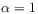, arise in physics, and why it arises more frequently than the case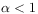.